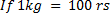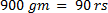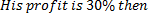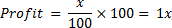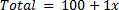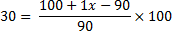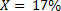## Profit and Loss For SBI PO Set – 1

1)  A company blends two varieties of tea from two different tea gardens, one variety costing Rs.20 per keg and other Rs.25 per kg, in the ratio 5:4. He sells the blended tea at Rs.23 per kg. Find his profit per cent?

a) 5%

b) 3.5 %

c) 3.5%

d)  No profit no loss

e) None of these

b) 3.5 %

Explanation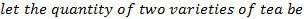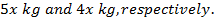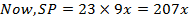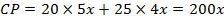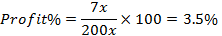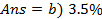2) A draper buys 100 shawls for Rs. 2,450. He sells 76 of them at Rs. 35 each, and the rest at half of that price. How much does he gain? What is his gain per cent on the cost price?

a) Rs. 630, 25 5/7%

b) Rs. 50, 26 26/49%

c) Rs. 730, 29 39/49 %

d) Rs. 832, 29 ¼%

e) None of these

a) Rs. 630, 25 5/7%

Explanation

C.P. of 100 shawls = Rs. 2450

S.P. of 100 shawls = 76 * 35 + 24* 35/2= 2660 + 420 =  Rs. 3080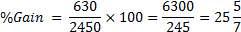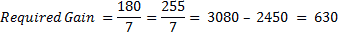3)  If books bought at prices ranging from Rs. 227 to Rs. 350 are sold at prices ranging from Rs. 300 to Rs. 428, what is the greatest possible profit that might be made in selling eighteen books?

a) 2648

b) 3618

c) 4816

d) 4536

e) None of these

b) 3618

Explanation

Least cost price = Rs. (227×18) = Rs.4086

Greatest selling price = Rs. (428×18 ) = 7704

Required profit = Rs. (7704-4086) = Rs. 3618

4) M, N and O jointly thought of engaging themselves in a business venture. It was agreed that M would invest Rs. 6500 for 6 months and N would invest Rs. 8400 for 5 months and O, Rs. 10000 for 3 months. M wants to be the working member for which, he was to receive 5% of the profit. The profit earned was Rs. 7400. Calculate the share of N in the profit (Rs. In approximately)?

a) 2880

b) 3556

c) 2660

d) 2940

e) None of these

c) 2660

Explanation

M received = 5% of Rs. 7400= Rs. 370

Balance= Rs. (7400-370)= Rs. 7030

Ratio of their investments(M:N:O)= (6500 *6):(8400*5 ):(10000 * 3)

=39000:42000:30000

=13:14:10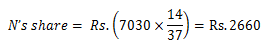5) A trader wants 15% profit on the selling price of a product whereas his expenses amount to 20% on sales. What should be his rate of mark up on article costing Rs.7.8? (Approximate)

a) 51 %

b) 62 %

c) 64 %

d) 54 %

e) None of these

d) 54 %

Explanation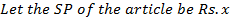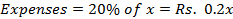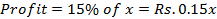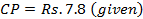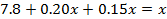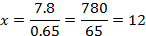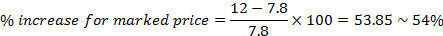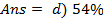6)  Mangoes were purchased at Rs.150 per hundred and sold at Rs.2per mango. If 2000 Mangoes were sold, what was the total profit does he make?

a) Rs.1200

b) Rs.1000

c) Rs.1400

d) Rs.2000

d) None of these

b) Rs.1000

Explanation

C. P for one Mango = Rs 150/100 = Rs.3/2

S. P for one Mango = Rs. 2

Profit on one Mango =Rs. 2 – 3/2 = Rs.1/2

Profit on 2000 Mangoes = 1/2 *2000

Profit on 2000 Mangoes = Rs. 1000

7) A trader has 320 kg of tea, a part of which he sells at 18% profit and the rest at 26% profit. He gains 21% gain on the whole. What is the quantity sold at 18% profit gain?

a) 215 kg

b) 200 kg

c) 120kg

d) 165 kg

e) 155 kg

b) 200 kg

Explanation

By the rule of allegation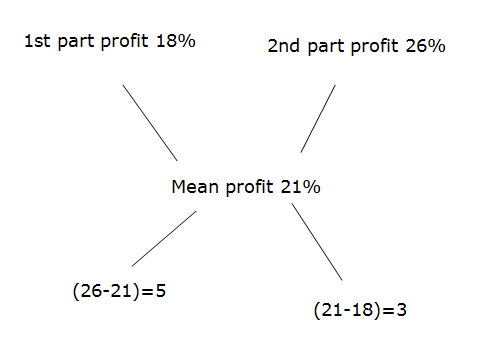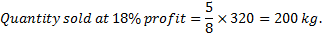8) Ninad, Vikas and Manav enter into a partnership. Ninad invests some amount at the beginning. Vikas invests double the amount after 6 months and Manav invests thrice the amount invested by Ninad after 8 months. They earn a profit of Rs. 45000 at the end of the year. What is Manav’s share in the profit?

a)  Rs. 25000

b)  Rs. 15000

c)  Rs. 12000

d)  Rs. 9000

e)  None of these

b)  Rs. 15000

Explanation

x × 12       2x × 6       3x × 4

x      :       x      :       x

3x = 45000

Manav’s profit = 15000

9) A dishonest trader cheats while buying from wholesaler and also while selling to a customer. While he takes 1200 gm per kg from wholesaler, he gives 900gm per kg to the customer. Also it is known that his mark up % and discount % is 60% and 10% respectively. Find his actual profit.

a) 72%

b) 85%

c) 92%

d) 100%

e) None of these

c) 92%

Explanation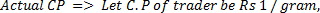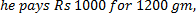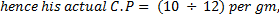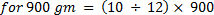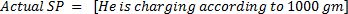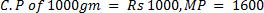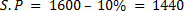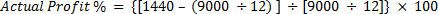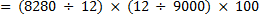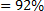10) A dishonest trader gives only 900 gm for 1 kg. Although he claims to be selling at a profit of x% above cost price, his actual Profit (owing to faulty measure) is 30%. What is the value of x?

a) 15

b) 16

c) 17

d) 21

e) 13

b) 16

Explanation